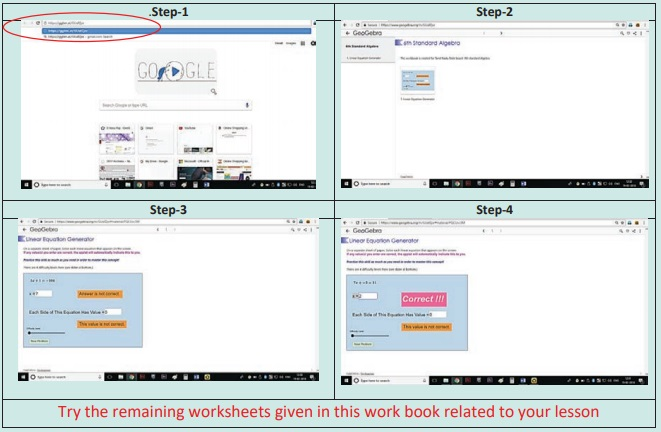Home | | Maths 6th Std | Summary

# Summary

6th Maths : Term 1 Unit 2 : Introduction To Algebra : Summary

Summary

Variables are quantities that can take any value and they are denoted by small alphabets a,b,c, …, x,y,z.

A Variable allows us to express relations easily in all practical situations.

Variables are used to generalise and express many common rules of Geometry and Arithmetic.

ICT Corner

INTRODUCTION TO ALGEBRA

Expected Result is shown in this pictureStep – 1

Open the Browser and copy and paste the Link given below (or) by typing the URL given (or) Scan the QR Code.

Step − 2

GeoGebra Work Book “6th Standard Algebra” will appear. There are several worksheets. In that open “Linear Equation Generator

Step-3

In the page select the difficulty level by moving the slider. Linear equation will appear on the top solve it and enter your answer in the “x” box and hit enter.

Step-4

If your answer is correct “Correct!!!” menu will appear. Try more problems by clicking on “New ProblemTry the remaining worksheets given in this work book related to your lesson